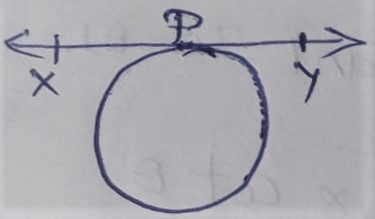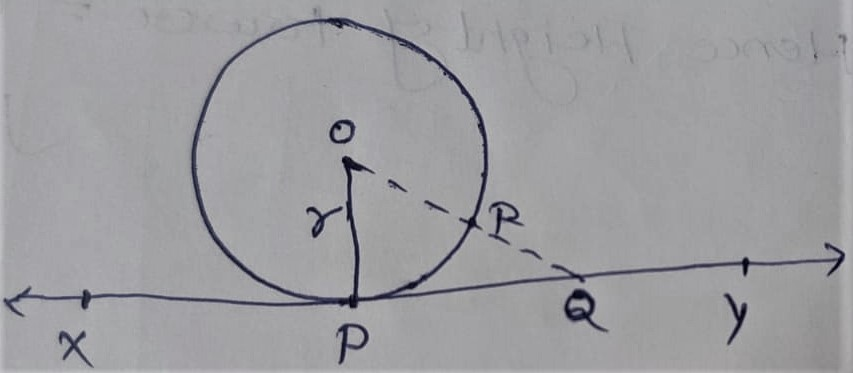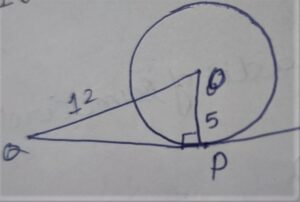# NCERT Circles Class 10 Exercise 10.1 Solution

Tangent to a Circle : a tangent to a circle is a line which touches or intersect the circle at only one point.• A tangent to a circle is a special type of secant ,In which both intersect points of secant are coincide.

Theorem 10.1

Prove that ” The tangent at any point of a circle is perpendicular to the radius through the of contact.”

Given:

C ( 0,r)X Y = tangent

P = point of contact

To Prove:

O P ⊥ (perpendicular) on X Y .

Construction:

• Take an another point Q  on tangent X Y Except ‘ P ‘
• Joint Q with center O
• O Q intersect the circle at ‘ R ‘

Proof:

In circle:

O P = O R { Radii of same circle } ………….equation(i)

O Q = O R + R Q

O Q > O R { by construction }

∴ O Q > O P { From equation ( i ) }

We can say that all point on tangent are not touches the circle i.e. exterior from the circle only P is touches the circle on tangent which is shortest distance from center O of the circle to the tangent

• Shortest distance is always perpendicular from a point to al line

∴ O P ⊥ ( perpendicular ) on  X Y .              Hence Proved

Key Point : Radius is always perpendicular on point of contact to the tangent.

## Exercise – 10.1

Q. 1. How many tangents can a circle have ?

Diagram

Q. 2. Fill in the blanks

( i )  A tangent to a circle intersects it in ……….. point(s)

Ans – One

(ii) A line intersecting a circle in two points is called a …………

Ans – Secant

(iii) A circle can have  ………… parallel tangents at the most.

Ans –  Two

(iv) The common point of a tangent to a circle and the circle is called ……..

Ans- Point of Contact

Q. 3. A tangent P Q at a point P of a circle of radius 5 cm meets a line through the center O at a point Q so that

O Q = 12cm . Length P Q is :

(A) 12cm

(B) 13cm

(C) 8.5cm

(D) √119cm

Ans- (D)

Explanation:

According to figureIn Δ OPQ

∠P = 90º

By PGT

(OQ)² = (OP)² + (PQ)²

=> (12)² = (5)² + (PQ)²

=> 144 = 25 + (PQ)²

=> 144 – 25 = (PQ)²

=> 119 = (PQ)²

=> √119 = PQ